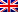#[EasyCTF 2018] [Reverse 150 – Soupstitution Cipher] Write Up

## Description

For this challenge, we need to reverse a briefly obfuscated python script to find an input value that will validate some conditions to get the flag.

```#!/usr/bin/env python3

from binascii import unhexlify as sOup
from operator import attrgetter as souP

ME_FLAGE = 'censored'

SoUp = input
soUP = hex
sOUp = print
sOuP = ord
SOuP = open

def SoUP(sOUP):
soup = 0
while sOUP != 0:
soup = (soup * 10) + (sOUP % 10)
sOUP //= 10
return soup

def SOup(sOUP):
soup = 0
for soUp in sOUP:
soup *= 10
soup += sOuP(soUp) - sOuP('0')
return soup

def SOUP():
Soup = SoUp()[:7]
print(Soup)
if not souP('isdigit')(Soup)():
sOUp("that's not a number lol")
return

soup = SoUP(SOup(Soup))
SouP = souP('zfill')(soUP(soup)[2:])(8)[-8:]
if sOup(SouP) == souP('encode')('s0up')():
sOUp("oh yay it's a flag!", ME_FLAGE)
else:
sOUp('oh noes rip u')

if __name__ == '__main__':
SOUP()

```

## Resolution

We first tried to understand what does the defined functions do:

• “SOUP” is the main function, it will read a line from stdin, do some stuff and finally give the flag or print an error message.
• “SoUP” takes an integer and reverses it. E.G.: if we give 1234 as input, it will return 4321.
• “SOup” takes a numerical string and converts it to an integer, like C function “atoi”.

Then, we took a closer eye on the condition to get the flag:

```# obfuscated
soup = SoUP(SOup(Soup))
SouP = souP('zfill')(soUP(soup)[2:])(8)[-8:]
if sOup(SouP) == souP('encode')('s0up')()

# unobfuscated
reversedInt = reverse(atoi(inputString))
hexNumericString = hex(reversedInt)[2:].zfill(8)[-8:]
if binascii.unhexlify(hexNumericString) == 's0up'.encode()
```

If we read the code backwords:

• “hexNumericString” must be equal to “73307570”
• “reversedInt” must be equal to the base 10 view of the previous number, aka “1932555632”
• “inputString” must be equal to the reverse value of the previous number, aka “2365552391”

We now have the number to validate the challenge ! … But it won’t work as the input length is limited to 7 characters and “2365552391” has 10 digits.

To bypass this limit, we need that “str.isdigit()” method returns true with other characters than 0-9 (0x30 – 0x39). And that’s the case, if we check all the characters from 0x0 to 0xffff, there is a lot of multibytes chars that are considered as digits by python:

```charset = list(filter(lambda x: x.isdigit(), map(chr, range(0x10000))))
print(charset)
```

Here’s an example considered as a valid digital string to python:

```digitalString = b'\xef\xbc\x99\xef\xbc\x98\xef\xbc\x97\xef\xbc\x96\xef\xbc\x95\xef\xbc\x94\xef\xbc\x93'.decode('utf-8')
print(len(digitalString)) # output: 7
print(digitalString.isdigit()) # output: True
```

By the way, giving such a string to the “atoi” function will result on much bigger numbers than the previous “9999999” limit.

We only had to find a string with our charset that will produce “2365552391” as output to the “atoi” function (Don’t forget that this function subtract 0x30 to each character before adding them to the sum). We found the last 4 characters manually by checking that ( sum – character – 0x30 ) was divisible by 10:

• sum = 2365552391, str = 65299. Next step: (2365552391 – 65299 – 48) / 10 =  236548714
• sum = 236548714, str = 65302. Next step: (236548714 – 65302 – 48) / 10 =  23648346
• sum = 23648346, str = 65304. Next step: (23648346 – 65304 – 48) / 10 =  2358309
• sum = 2358309, str = 65297. Next step: (2358309 – 65297 – 48) / 10 = 229306

Finally, we bruteforced the last 3 characters to find the expected sum. We found that the following numbers matched the conditions: 1991, 2541, 10124, 65297, 65304, 65302, 65299; which are represented by the string b’\xdf\x87\xe0\xa7\xad\xe2\x9e\x8c\xef\xbc\x91\xef\xbc\x98\xef\xbc\x96\xef\xbc\x93′

As it was working locally, we passed it to the network validation service:

```\$ echo \$'\xdf\x87\xe0\xa7\xad\xe2\x9e\x8c\xef\xbc\x91\xef\xbc\x98\xef\xbc\x96\xef\xbc\x93' | nc c1.easyctf.com 12484
߇৭➌１８６３
oh yay it's a flag! easyctf{S0up_soup_soUP_sOuP_s0UP_S0up_s000000OOOOOOuuuuuuuuppPPppPPPp}
```

Flag was easyctf{S0up_soup_soUP_sOuP_s0UP_S0up_s000000OOOOOOuuuuuuuuppPPppPPPp}